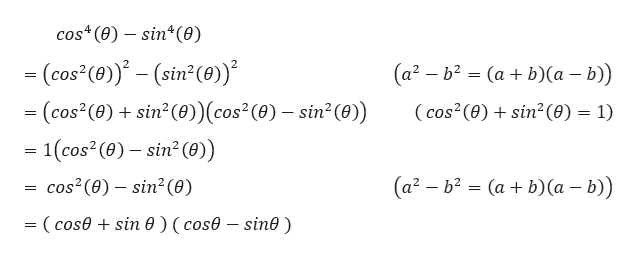# Simplify the following showing steps: Cos4(theta)-Sin4(theta)

Question
31 views

Simplify the following showing steps:

Cos4(theta)-Sin4(theta)

check_circle

Step 1

Given,

...help_outlineImage Transcriptionclosecos (e) sin (0) (cos (0)-(sin (0) - (cos (e)+ sin (0)(cos (e)- stn (0) = 1(cos2 (e)- sin2 (0) (a2 b2 (ab) (a - b)) (cos2 (e)sin2 (0) 1) = = (a2 b2(ab) (a - b)) cos2 (0) sin2 (e) - (cosesin e ) (cose - sin0) fullscreen

### Want to see the full answer?

See Solution

#### Want to see this answer and more?

Solutions are written by subject experts who are available 24/7. Questions are typically answered within 1 hour.*

See Solution
*Response times may vary by subject and question.
Tagged in

### Trigonometry